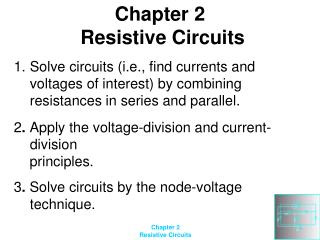DownloadDownload PresentationChapter 2 Resistive Circuits

# Chapter 2 Resistive Circuits

Download Presentation## Chapter 2 Resistive Circuits

- - - - - - - - - - - - - - - - - - - - - - - - - - - E N D - - - - - - - - - - - - - - - - - - - - - - - - - - -
##### Presentation Transcript

1. Chapter 2 Resistive Circuits • Solve circuits (i.e., find currents and voltages of interest) by combining resistances in series and parallel. • 2. Apply the voltage-division and current-division • principles. • 3. Solve circuits by the node-voltage technique.

2. 4.Find Thévenin and Norton equivalents. 5.Apply the superposition principle. 6.Draw the circuit diagram and state the principles of operation for the Wheatstone bridge.

3. Begin by locating a combination of resistances that are in series or parallel. Often the place to start is farthest from the source. Redraw the circuit with the equivalent resistance for the combination found in step 1. Circuit Analysis using Series/Parallel Equivalents

4. 3. Repeat steps 1 and 2 until the circuit is reduced as far as possible. Often (but not always) we end up with a single source and a single resistance. 4. Solve for the currents and voltages in the final equivalent circuit.

5. Voltage Division

6. Application of the Voltage-Division Principle

7. Current Division

8. Application of the Current-Division Principle

9. Although they are veryimportant concepts,series/parallel equivalents andthe current/voltage divisionprinciples are not sufficient tosolve all circuits.

10. Node Voltage Analysis

11. Circuits with Voltage Sources We obtain dependentequations if we use all of thenodes in a network to writeKCL equations.

12. Node-Voltage Analysis with a Dependent SourceFirst, we write KCL equations at each node, including the current of the controlled source just as if it were an ordinary current source.

13. Next, we find an expression for the controlling variable ixin terms of the node voltages.

14. Substitution yields

15. Node-Voltage Analysis 1. Select a reference node and assign variables for the unknown node voltages. If the reference node is chosen at one end of an independent voltage source, one node voltage is known at the start, and fewer need to be computed.

16. 2. Write network equations. First, use KCL to write current equations for nodes and supernodes. Write as many current equations as you can without using all of the nodes. Then if you do not have enough equations because of voltage sources connected between nodes, use KVL to write additional equations.

17. 3. If the circuit contains dependent sources, find expressions for the controlling variables in terms of the node voltages. Substitute into the network equations, and obtain equations having only the node voltages as unknowns.

18. 4. Put the equations into standard form and solve for the node voltages. 5. Use the values found for the node voltages to calculate any other currents or voltages of interest.